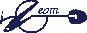Lessons with iGeomInteractive Geometry - cronological order
 Lessons

iGeom

This is an exemple of how to introduce Interactive Geometry with iGeom. Its target are classes of students that already know the basic constructions of Geometry, for instance, how to construct a midpoint using ruler and compass.

This material has been used at LEM with K-12 math students and teachers.

 Lessons Subjects Lesson 1 Introduction to iGeom and interactive geometry Lesson 2 Basic constructions related to notable point: angles, midpoint, perpendicular bisector; bisector; right triangle; square quadrilateral. Lesson 3 Minimal distance: problems which solution use the isometries reflection and translation Lesson 4 Minimal distance: problem which solution use the rotation isometry Lesson 5 Classical notable points: centroid; circumcenter; orthocenter; incenter. Lesson 6 Extensions of notable points: Ceva's theorem; Euler's line and Napoleon's theorem.

Observation: iGeom do not use the "drag-and-drop" pattern, in order to reduce stress-related muscle tension, reducing harm with the intensive use of the software. So, usually you need to click the construction button, them click the drawing area (or some object).

 iMática IME USP